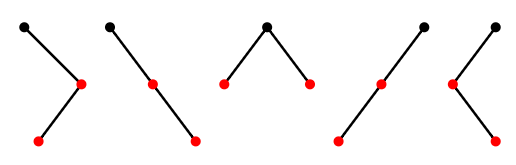$$\newcommand{\id}{\mathrm{id}}$$ $$\newcommand{\Span}{\mathrm{span}}$$ $$\newcommand{\kernel}{\mathrm{null}\,}$$ $$\newcommand{\range}{\mathrm{range}\,}$$ $$\newcommand{\RealPart}{\mathrm{Re}}$$ $$\newcommand{\ImaginaryPart}{\mathrm{Im}}$$ $$\newcommand{\Argument}{\mathrm{Arg}}$$ $$\newcommand{\norm}{\| #1 \|}$$ $$\newcommand{\inner}{\langle #1, #2 \rangle}$$ $$\newcommand{\Span}{\mathrm{span}}$$

# 3.6: Catalan Numbers

•• Contributed by David Guichard
• Professor (Mathematics) at Whitman College
$$\newcommand{\vecs}{\overset { \rightharpoonup} {\mathbf{#1}} }$$ $$\newcommand{\vecd}{\overset{-\!-\!\rightharpoonup}{\vphantom{a}\smash {#1}}}$$$$\newcommand{\id}{\mathrm{id}}$$ $$\newcommand{\Span}{\mathrm{span}}$$ $$\newcommand{\kernel}{\mathrm{null}\,}$$ $$\newcommand{\range}{\mathrm{range}\,}$$ $$\newcommand{\RealPart}{\mathrm{Re}}$$ $$\newcommand{\ImaginaryPart}{\mathrm{Im}}$$ $$\newcommand{\Argument}{\mathrm{Arg}}$$ $$\newcommand{\norm}{\| #1 \|}$$ $$\newcommand{\inner}{\langle #1, #2 \rangle}$$ $$\newcommand{\Span}{\mathrm{span}}$$ $$\newcommand{\id}{\mathrm{id}}$$ $$\newcommand{\Span}{\mathrm{span}}$$ $$\newcommand{\kernel}{\mathrm{null}\,}$$ $$\newcommand{\range}{\mathrm{range}\,}$$ $$\newcommand{\RealPart}{\mathrm{Re}}$$ $$\newcommand{\ImaginaryPart}{\mathrm{Im}}$$ $$\newcommand{\Argument}{\mathrm{Arg}}$$ $$\newcommand{\norm}{\| #1 \|}$$ $$\newcommand{\inner}{\langle #1, #2 \rangle}$$ $$\newcommand{\Span}{\mathrm{span}}$$

A rooted binary tree is a type of graph that is particularly of interest in some areas of computer science. A typical rooted binary tree is shown in Figure $$\PageIndex{1}$$ . The root is the topmost vertex. The vertices below a vertex and connected to it by an edge are the children of the vertex. It is a binary tree because all vertices have 0, 1, or 2 children. How many different rooted binary trees are there with $$n$$ vertices?Figure $$\PageIndex{1}$$: A rooted binary tree.

Let us denote this number by $$C_n$$; these are the Catalan numbers. For convenience, we allow a rooted binary tree to be empty, and let $$C_0=1$$. Then it is easy to see that $$C_1=1$$ and $$C_2=2$$, and not hard to see that $$C_3=5$$. Notice that any rooted binary tree on at least one vertex can be viewed as two (possibly empty) binary trees joined into a new tree by introducing a new root vertex and making the children of this root the two roots of the original trees; see Figure $$\PageIndex{1}$$. (To make the empty tree a child of the new vertex, simply do nothing, that is, omit the corresponding child.)Figure $$\PageIndex{1}$$: Producing a new tree from smaller trees.

Thus, to make all possible binary trees with $$n$$ vertices, we start with a root vertex, and then for its two children insert rooted binary trees on $$k$$ and $$l$$ vertices, with $$k+l=n-1$$, for all possible choices of the smaller trees. Now we can write

$$C_n=\sum_{i=0}^{n-1} C_iC_{n-i-1}.$$

For example, since we know that $$C_0=C_1=1$$ and $$C_2=2$$,

$$C_3 = C_0C_2 + C_1C_1+C_2C_0 = 1\cdot2 + 1\cdot1 + 2\cdot1 = 5,$$

as mentioned above. Once we know the trees on 0, 1, and 2 vertices, we can combine them in all possible ways to list the trees on 3 vertices, as shown in Figure $$\PageIndex{3}$$. Note that the first two trees have no left child, since the only tree on 0 vertices is empty, and likewise the last two have no right child.Figure $$\PageIndex{3}$$:The 3-vertex binary rooted trees.

Now we use a generating function to find a formula for $$C_n$$. Let $$f=\sum_{i=0}^\infty C_ix^i$$. Now consider $$f^2$$: the coefficient of the term $$x^n$$ in the expansion of $$f^2$$ is $$\sum_{i=0}^{n} C_iC_{n-i}$$, corresponding to all possible ways to multiply terms of $$f$$ to get an $$x^n$$ term: $$C_0\cdot C_nx^n + C_1x\cdot C_{n-1}x^{n-1} + C_2x^2\cdot C_{n-2}x^{n-2} +\cdots+C_nx^n\cdot C_0.$$ Now we recognize this as precisely the sum that gives $$C_{n+1}$$, so $$f^2 = \sum_{n=0}^\infty C_{n+1}x^n$$. If we multiply this by $$x$$ and add 1 (which is $$C_0$$) we get exactly $$f$$ again, that is, $$xf^2+1=f$$ or $$xf^2-f+1=0$$; here 0 is the zero function, that is, $$xf^2-f+1$$ is 0 for all x. Using the Pythagorean theorem,

$$f={1\pm\sqrt{1-4x}\over 2x},$$

as long as $$x\not=0$$. It is not hard to see that as $$x$$ approaches 0,

$${1+\sqrt{1-4x}\over 2x}$$

goes to infinity while

$${1-\sqrt{1-4x}\over 2x}$$

goes to 1. Since we know $$f(0)=C_0=1$$, this is the $$f$$ we want.

Now by Newton's Binomial Theorem, we can expand

$$\sqrt{1-4x} = (1+(-4x))^{1/2} =\sum_{n=0}^\infty {1/2\choose n}(-4x)^n.$$

Then

$${1-\sqrt{1-4x}\over 2x} = \sum_{n=1}^\infty -{1\over 2}{1/2\choose n}(-4)^nx^{n-1} = \sum_{n=0}^\infty -{1\over 2}{1/2\choose n+1}(-4)^{n+1}x^n.$$

Expanding the binomial coefficient $$1/2\choose n+1$$ and reorganizing the expression, we discover that

$$C_n = -{1\over 2}{1/2\choose n+1}(-4)^{n+1} = {1\over n+1}{2n\choose n}.$$

In exercise 7 in section 1.2, we saw that the number of properly matched sequences of parentheses of length $$2n$$ is $${2n\choose n}-{2n\choose n+1}$$, and called this $$C_n$$. It is not difficult to see that

$${2n\choose n}-{2n\choose n+1}={1\over n+1}{2n\choose n},$$

so the formulas are in agreement.

Temporarily let $$A_n$$ be the number of properly matched sequences of parentheses of length $$2n$$, so from the exercise we know $$A_n={2n\choose n}-{2n\choose n+1}$$. It is possible to see directly that $$A_0=A_1=1$$ and that the numbers $$A_n$$ satisfy the same recurrence relation as do the $$C_n$$, which implies that $$A_n=C_n$$, without manipulating the generating function.

There are many counting problems whose answers turns out to be the Catalan numbers. Enumerative Combinatorics: Volume 2, by Richard Stanley, contains a large number of examples.